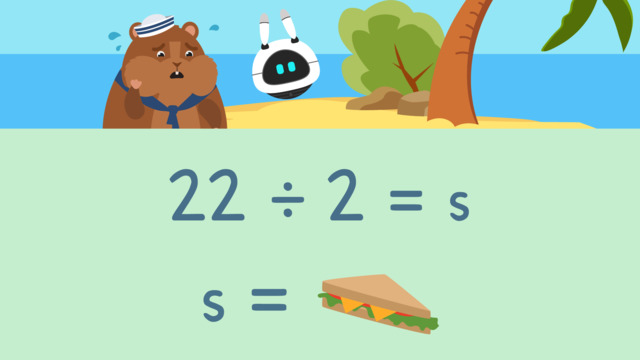# Solving Equations with Multiplication and DivisionRating

Be the first to give a rating!

The authorsTeam Digital
Solving Equations with Multiplication and Division
CCSS.MATH.CONTENT.4.OA.A.2

## Basics on the topicSolving Equations with Multiplication and Division

### Content

Mr. Squeaks and Imani are sailing on the open sea when all of a sudden, their boat takes a wrong turn and gets stuck on a deserted island! They don’t know how long they will be stuck so they want to make sure they have enough food. Let’s help them calculate the amount of food they have by solving equations using multiplication and division.

### One-step Multiplication & Division Equations from Word Problems

How do you solve one step equations with multiplication and division? How do you solve multiplication equations? Solving equations with multiplication and division is simple because it follows the basic rules of multiplication and division. However, before we can solve, we need to determine the equation based on the information from the word problem. The next section shows an example of one step equations with multiplication and division that come from a word problem.

#### Solving Equations Using Multiplication and Division from a Word Problem Example

The first step is to read the word problem. As you read, think; 'what do I need to find?', and highlight the question you need to solve!Next, highlight all the other important information.Now we need to identify the operation. The keywords in each means to divide in this problem because the sandwiches are being shared equally.Then, write the equation using a variable to represent the unknown value. A variable is a letter that we use to represent an unknown value. Our equation is: twenty-two sandwiches divided by two bags equals S because we are dividing the number of sandwiches they have into two bags. We use the letter S as the variable to represent the number of sandwiches.The last step is to solve the equation. We can draw pictures to help us solve, so here you see twenty-two sandwiches and two bags.What is twenty-two divided by two? Eleven, so they have eleven sandwiches in each bag. Write the answer using our variable: S equals eleven.### Solving Equations Using Multiplication and Division Summary

How do you solve equations by multiplication and division? Solving equations with multiplication and division is simple because it follows the basic rules of multiplication and division. However, before we can solve, we need to determine the equation based on the information from the word problem. In order to determine the equation, we follow the steps for solving a word problem:

• Step 1: Read the word problem. As you read, think; 'what do I need to find?', and highlight the question you need to solve

• Step 2: Highlight all the other important information

• Step 3: Identify the operation

• Step 4: Write the equation using a variable to represent the unknown value

• Step 5: Solve the equation. We can also draw pictures to help us solve

Want some more solving equations using multiplication and division practice? On this website you can find solving equations using multiplication and division worksheets and a solving equations using multiplication and division worksheet along with activities and exercises.